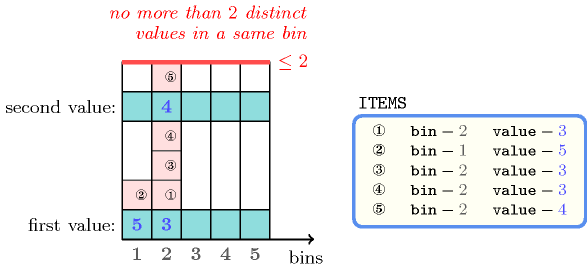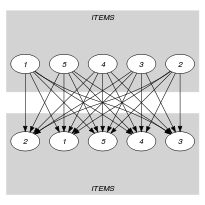## 5.35. assign_and_nvalues

Origin
Constraint

$\mathrm{𝚊𝚜𝚜𝚒𝚐𝚗}_\mathrm{𝚊𝚗𝚍}_\mathrm{𝚗𝚟𝚊𝚕𝚞𝚎𝚜}\left(\mathrm{𝙸𝚃𝙴𝙼𝚂},\mathrm{𝚁𝙴𝙻𝙾𝙿},\mathrm{𝙻𝙸𝙼𝙸𝚃}\right)$

Arguments
 $\mathrm{𝙸𝚃𝙴𝙼𝚂}$ $\mathrm{𝚌𝚘𝚕𝚕𝚎𝚌𝚝𝚒𝚘𝚗}\left(\mathrm{𝚋𝚒𝚗}-\mathrm{𝚍𝚟𝚊𝚛},\mathrm{𝚟𝚊𝚕𝚞𝚎}-\mathrm{𝚍𝚟𝚊𝚛}\right)$ $\mathrm{𝚁𝙴𝙻𝙾𝙿}$ $\mathrm{𝚊𝚝𝚘𝚖}$ $\mathrm{𝙻𝙸𝙼𝙸𝚃}$ $\mathrm{𝚍𝚟𝚊𝚛}$
Restrictions
 $\mathrm{𝚛𝚎𝚚𝚞𝚒𝚛𝚎𝚍}$$\left(\mathrm{𝙸𝚃𝙴𝙼𝚂},\left[\mathrm{𝚋𝚒𝚗},\mathrm{𝚟𝚊𝚕𝚞𝚎}\right]\right)$ $\mathrm{𝚁𝙴𝙻𝙾𝙿}\in \left[=,\ne ,<,\ge ,>,\le \right]$
Purpose

Given several items (each of them having a specific value that may not be initially fixed), and different bins, assign each item to a bin, so that the number $n$ of distinct values in each bin satisfies the condition $n\mathrm{𝚁𝙴𝙻𝙾𝙿}\mathrm{𝙻𝙸𝙼𝙸𝚃}$.

Example
$\left(\begin{array}{c}〈\begin{array}{cc}\mathrm{𝚋𝚒𝚗}-2\hfill & \mathrm{𝚟𝚊𝚕𝚞𝚎}-3,\hfill \\ \mathrm{𝚋𝚒𝚗}-1\hfill & \mathrm{𝚟𝚊𝚕𝚞𝚎}-5,\hfill \\ \mathrm{𝚋𝚒𝚗}-2\hfill & \mathrm{𝚟𝚊𝚕𝚞𝚎}-3,\hfill \\ \mathrm{𝚋𝚒𝚗}-2\hfill & \mathrm{𝚟𝚊𝚕𝚞𝚎}-3,\hfill \\ \mathrm{𝚋𝚒𝚗}-2\hfill & \mathrm{𝚟𝚊𝚕𝚞𝚎}-4\hfill \end{array}〉,\le ,2\hfill \end{array}\right)$

Figure 5.35.1 depicts the solution corresponding to the example.

##### Figure 5.35.1. An assignment with at most two distinct values in parallel (values 3 and 4 in bin 2 and value 5 in bin 1)The $\mathrm{𝚊𝚜𝚜𝚒𝚐𝚗}_\mathrm{𝚊𝚗𝚍}_\mathrm{𝚗𝚟𝚊𝚕𝚞𝚎𝚜}$ constraint holds since for each used bin (i.e., namely bins 1 and 2) the number of distinct colours of the corresponding assigned items is less than or equal to the limit 2.

Typical
 $|\mathrm{𝙸𝚃𝙴𝙼𝚂}|>1$ $\mathrm{𝚛𝚊𝚗𝚐𝚎}$$\left(\mathrm{𝙸𝚃𝙴𝙼𝚂}.\mathrm{𝚋𝚒𝚗}\right)>1$ $\mathrm{𝚛𝚊𝚗𝚐𝚎}$$\left(\mathrm{𝙸𝚃𝙴𝙼𝚂}.\mathrm{𝚟𝚊𝚕𝚞𝚎}\right)>1$ $\mathrm{𝚁𝙴𝙻𝙾𝙿}\in \left[<,\le \right]$ $\mathrm{𝙻𝙸𝙼𝙸𝚃}>1$ $\mathrm{𝙻𝙸𝙼𝙸𝚃}<|\mathrm{𝙸𝚃𝙴𝙼𝚂}|$
Symmetries
• Items of $\mathrm{𝙸𝚃𝙴𝙼𝚂}$ are permutable.

• All occurrences of two distinct values of $\mathrm{𝙸𝚃𝙴𝙼𝚂}.\mathrm{𝚋𝚒𝚗}$ can be swapped; all occurrences of a value of $\mathrm{𝙸𝚃𝙴𝙼𝚂}.\mathrm{𝚋𝚒𝚗}$ can be renamed to any unused value.

Arg. properties
• Contractible wrt. $\mathrm{𝙸𝚃𝙴𝙼𝚂}$ when $\mathrm{𝚁𝙴𝙻𝙾𝙿}\in \left[<,\le \right]$.

• Extensible wrt. $\mathrm{𝙸𝚃𝙴𝙼𝚂}$ when $\mathrm{𝚁𝙴𝙻𝙾𝙿}\in \left[\ge ,>\right]$.

Usage

Let us give two examples where the $\mathrm{𝚊𝚜𝚜𝚒𝚐𝚗}_\mathrm{𝚊𝚗𝚍}_\mathrm{𝚗𝚟𝚊𝚕𝚞𝚎𝚜}$ constraint is useful:

• Quite often, in bin-packing problems, each item has a specific type, and one wants to assign items of similar type to each bin.

• In a vehicle routing problem, one wants to restrict the number of towns visited by each vehicle. Note that several customers may be located at the same town. In this example, each bin would correspond to a vehicle, each item would correspond to a visit to a customer, and the colour of an item would be the location of the corresponding customer.

Keywords
Arc input(s)

$\mathrm{𝙸𝚃𝙴𝙼𝚂}$ $\mathrm{𝙸𝚃𝙴𝙼𝚂}$

Arc generator
$\mathrm{𝑃𝑅𝑂𝐷𝑈𝐶𝑇}$$↦\mathrm{𝚌𝚘𝚕𝚕𝚎𝚌𝚝𝚒𝚘𝚗}\left(\mathrm{𝚒𝚝𝚎𝚖𝚜}\mathtt{1},\mathrm{𝚒𝚝𝚎𝚖𝚜}\mathtt{2}\right)$

Arc arity
Arc constraint(s)
$\mathrm{𝚒𝚝𝚎𝚖𝚜}\mathtt{1}.\mathrm{𝚋𝚒𝚗}=\mathrm{𝚒𝚝𝚎𝚖𝚜}\mathtt{2}.\mathrm{𝚋𝚒𝚗}$
Graph class
 $•$$\mathrm{𝙰𝙲𝚈𝙲𝙻𝙸𝙲}$ $•$$\mathrm{𝙱𝙸𝙿𝙰𝚁𝚃𝙸𝚃𝙴}$ $•$$\mathrm{𝙽𝙾}_\mathrm{𝙻𝙾𝙾𝙿}$

Sets
$\begin{array}{c}\mathrm{𝖲𝖴𝖢𝖢}↦\hfill \\ \left[\begin{array}{c}\mathrm{𝚜𝚘𝚞𝚛𝚌𝚎},\hfill \\ \mathrm{𝚟𝚊𝚛𝚒𝚊𝚋𝚕𝚎𝚜}-\mathrm{𝚌𝚘𝚕}\left(\begin{array}{c}\mathrm{𝚅𝙰𝚁𝙸𝙰𝙱𝙻𝙴𝚂}-\mathrm{𝚌𝚘𝚕𝚕𝚎𝚌𝚝𝚒𝚘𝚗}\left(\mathrm{𝚟𝚊𝚛}-\mathrm{𝚍𝚟𝚊𝚛}\right),\hfill \\ \mathrm{𝚒𝚝𝚎𝚖}\left(\mathrm{𝚟𝚊𝚛}-\mathrm{𝙸𝚃𝙴𝙼𝚂}.\mathrm{𝚟𝚊𝚕𝚞𝚎}\right)\right]\hfill \end{array}\right)\hfill \end{array}\right]\hfill \end{array}$

Constraint(s) on sets
$\mathrm{𝚗𝚟𝚊𝚕𝚞𝚎𝚜}$$\left(\mathrm{𝚟𝚊𝚛𝚒𝚊𝚋𝚕𝚎𝚜},\mathrm{𝚁𝙴𝙻𝙾𝙿},\mathrm{𝙻𝙸𝙼𝙸𝚃}\right)$
Graph model

We enforce the $\mathrm{𝚗𝚟𝚊𝚕𝚞𝚎𝚜}$ constraint on the items that are assigned to the same bin.

Parts (A) and (B) of Figure 5.35.2 respectively show the initial and final graph associated with the Example slot. The final graph consists of the following two connected components:

• The connected component containing 8 vertices corresponds to the items that are assigned to bin 2.

• The connected component containing 2 vertices corresponds to the items that are assigned to bin 1.

##### Figure 5.35.2. Initial and final graph of the $\mathrm{𝚊𝚜𝚜𝚒𝚐𝚗}_\mathrm{𝚊𝚗𝚍}_\mathrm{𝚗𝚟𝚊𝚕𝚞𝚎𝚜}$ constraint(a) (b)

The $\mathrm{𝚊𝚜𝚜𝚒𝚐𝚗}_\mathrm{𝚊𝚗𝚍}_\mathrm{𝚗𝚟𝚊𝚕𝚞𝚎𝚜}$ constraint holds since for each set of successors of the vertices of the final graph no more than two distinct values are used:

• The unique item assigned to bin 1 uses value 5.

• Items assigned to bin 2 use values 3 and 4.